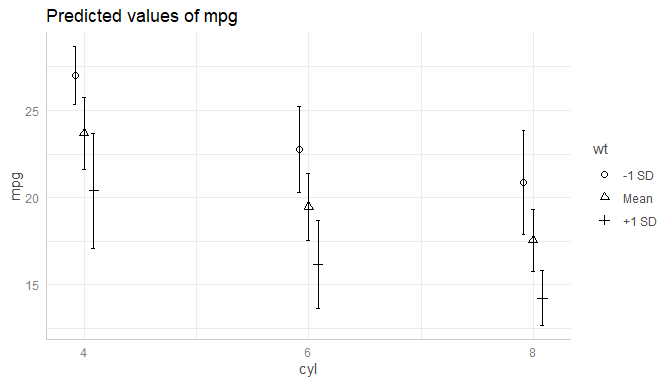# Introduction: Customize Plot Appearance

#### 2020-03-14

This vignettes demonstrates how to customize plots created with the plot()-method of the ggeffects-package.

plot() returns an object of class ggplot, so it is easy to apply further modifications to the resulting plot. You may want to load the ggplot2-package to do this: library(ggplot2).

library(ggeffects)
library(ggplot2)

data(mtcars)
m <- lm(mpg ~ gear + as.factor(cyl) + wt, data = mtcars)

# continuous x-axis
dat <- ggpredict(m, terms = c("gear", "wt"))

# discrete x-axis
dat_categorical <- ggpredict(m, terms = c("cyl", "wt"))

# default plot
plot(dat)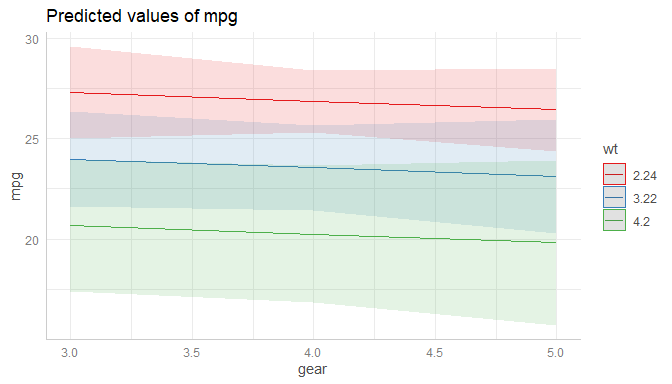## Changing Plot and Axis Titles

The simplest thing is to change the titles from the plot, x- and y-axis. This can be done with ggplot2::labs():

plot(dat) +
labs(
x = "Number of forward gears",
y = "Miles/(US) gallon",
title = "Predicted mean miles per gallon"
)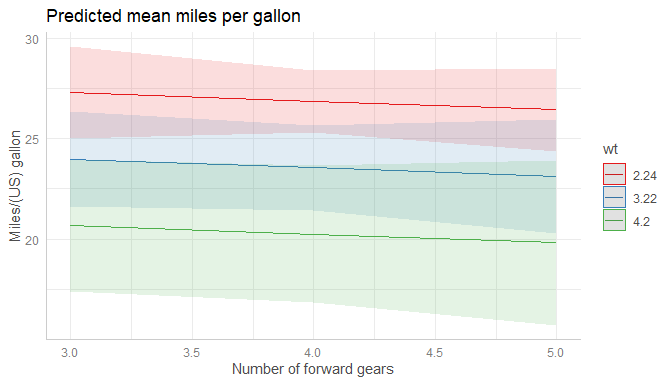## Changing the Legend Title

The legend-title can also be changed using the labs()-function. The legend in ggplot-objects refers to the aesthetic used for the grouping variable, which is by default the colour, i.e. the plot is constructed in the following way:

ggplot(data, aes(x = x, y = predicted, colour = group))

### Plots with Default Colors

Hence, using colour in labs() changes the legend-title:

plot(dat) + labs(colour = "Weight (1000 lbs)")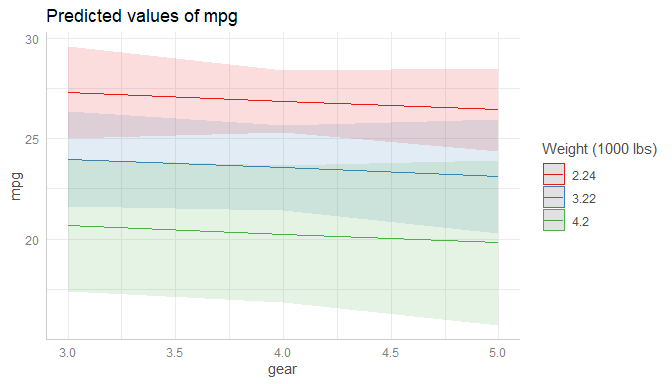### Black-and-White Plots

For black-and-white plots, the group-aesthetic is mapped to different linetypes, not to different colours. Thus, the legend-title for black-and-white plots can be changed using linetype in labs():

plot(dat, colors = "bw") + labs(linetype = "Weight (1000 lbs)")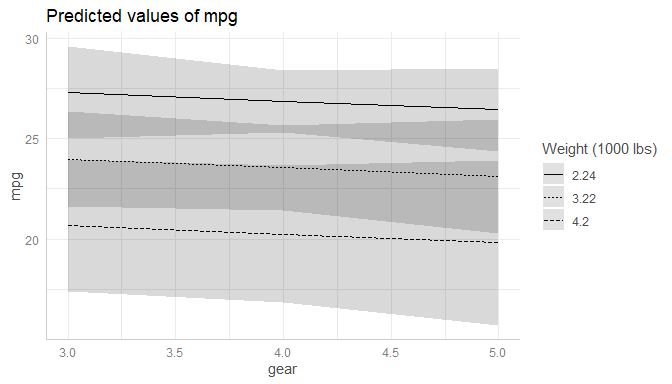### Black-and-White Plots with Categorical Predictor

If the variable on the x-axis is discrete for a black-and-white plot, the group-aesthetic is mapped to different shapes, so following code must be used to change the legend title:

plot(dat_categorical, colors = "bw") + labs(shape = "Weight (1000 lbs)")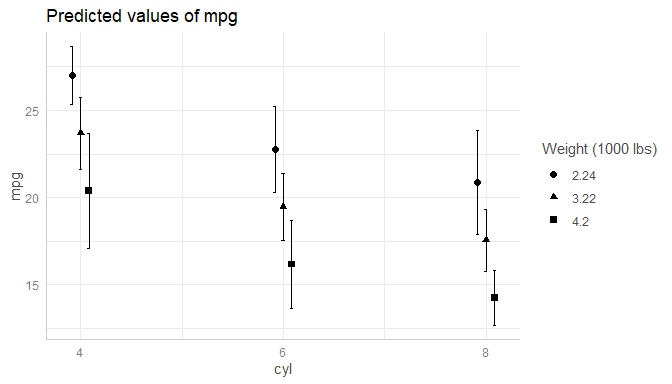## Changing the x-Axis Appearance

The x-axis for plots returned from plot() is always continuous, even for discrete x-axis-variables. The reason for this is that many users are used to plots that connect the data points with lines, which is only possible for continuous x-axes. You can do this using the connect.lines-argument:

plot(dat_categorical, connect.lines = TRUE)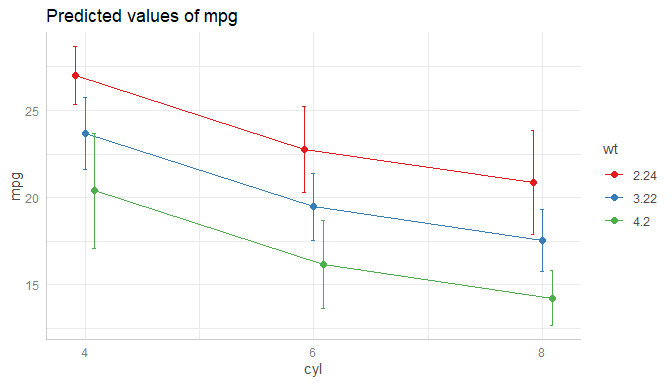### Categorical Predictors

Since the x-axis is continuous (i.e. ggplot2::scale_x_continuous()), you can use scale_x_continuous() to modify the x-axis, and change breaks, limits or labels.

plot(dat_categorical) +
scale_x_continuous(labels = c("four", "six", "eight"), breaks = c(4, 6, 8))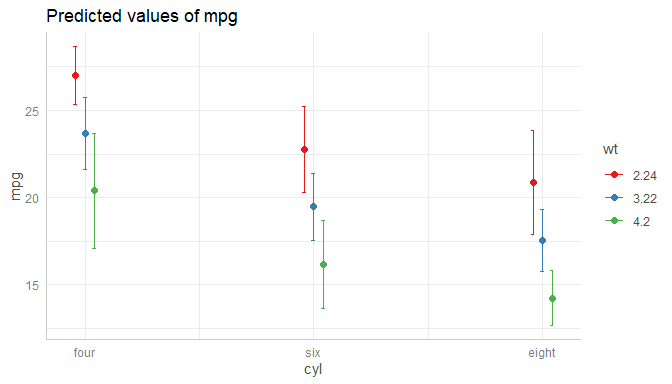### Continuous Predictors

Or for continuous variables:

plot(dat) + scale_x_continuous(breaks = 3:5, limits = c(2, 6))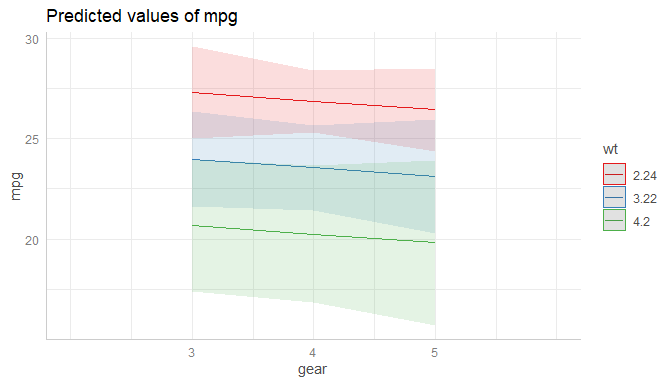## Changing the y-Axis Appearance

Arguments in ... are passed down to ggplot::scale_y_continuous() (resp. ggplot::scale_y_log10(), if log.y = TRUE), so you can control the appearance of the y-axis by putting the arguments directly into the call to plot():

plot(dat_categorical, breaks = seq(12, 30, 2), limits = c(12, 30))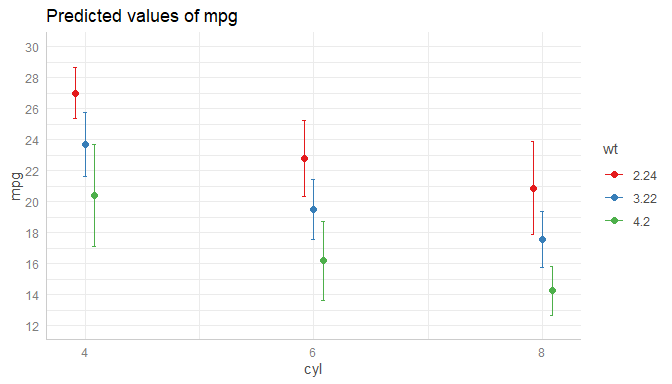## Changing the Legend Labels

The legend labels can also be changed using a scale_*()-function from ggplot. Depending on the color-setting (see section Changing the Legend Title), following functions can be used to change the legend labels:

• scale_colour_manual() resp. scale_colour_brewer()
• scale_linetype_manual()
• scale_shape_manual()

Since you overwrite an exising “color” scale, you typically need to provide the values or palette-argument, to manuall set the colors, linetypes or shapes.

### Plots with Default Colors

For plots using default colors:

plot(dat) +
scale_colour_brewer(palette = "Set1", labels = c("-1 SD", "Mean", "+1 SD"))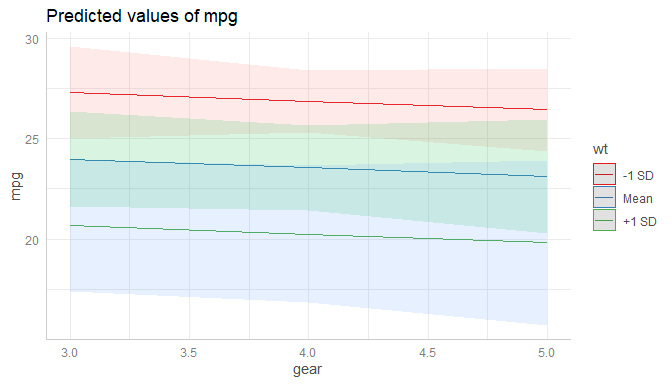### Black-and-White Plots

For black-and-white plots:

plot(dat, colors = "bw") +
scale_linetype_manual(values = 15:17, labels = c("-1 SD", "Mean", "+1 SD"))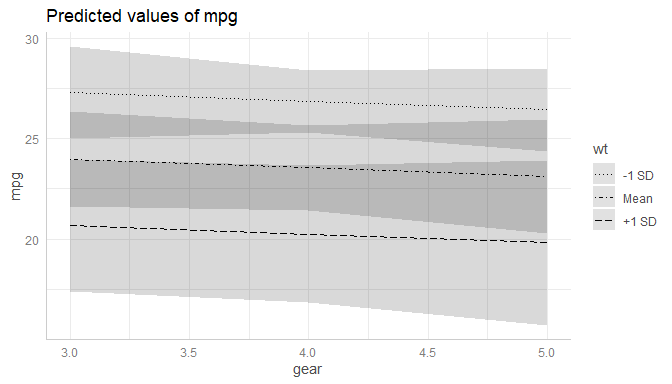### Black-and-White Plots with Categorical Predictor

For black-and-white plots with categorical x-axis:

plot(dat_categorical, colors = "bw") +
scale_shape_manual(values = 1:3, labels = c("-1 SD", "Mean", "+1 SD"))